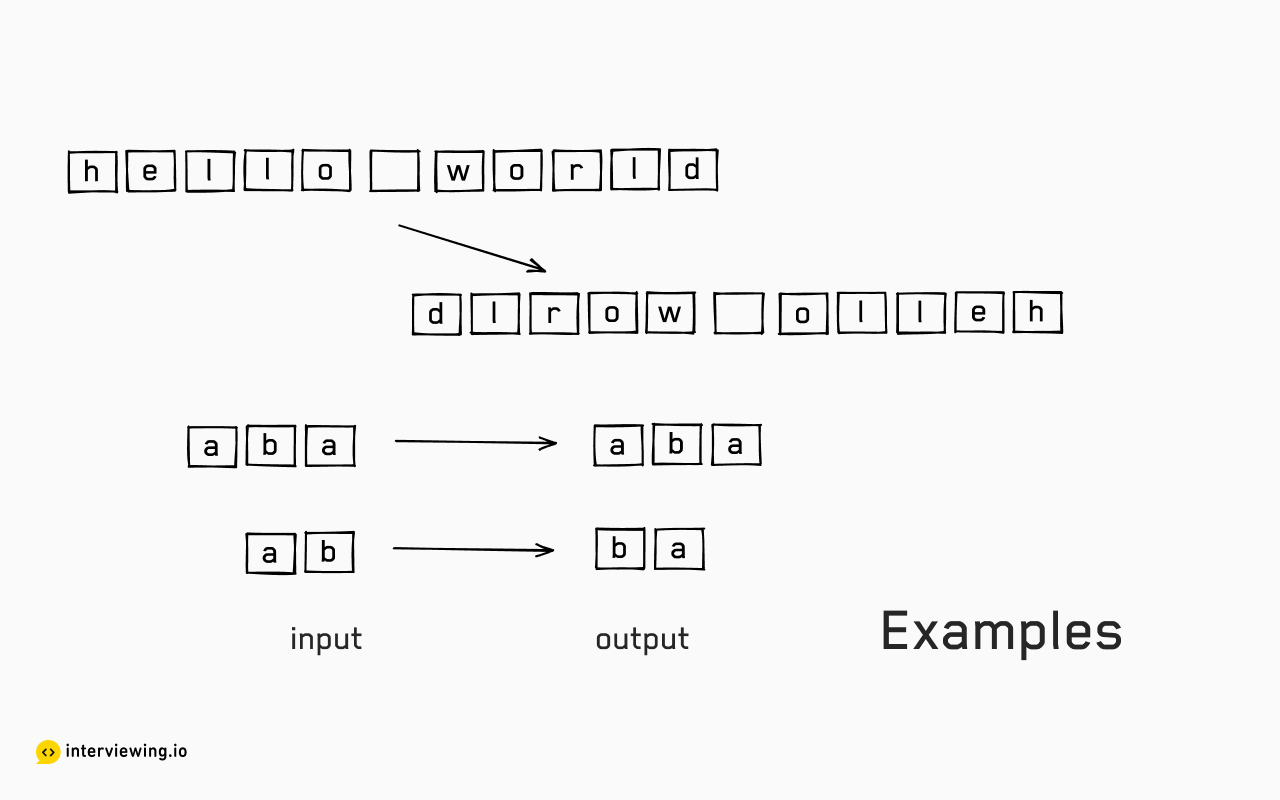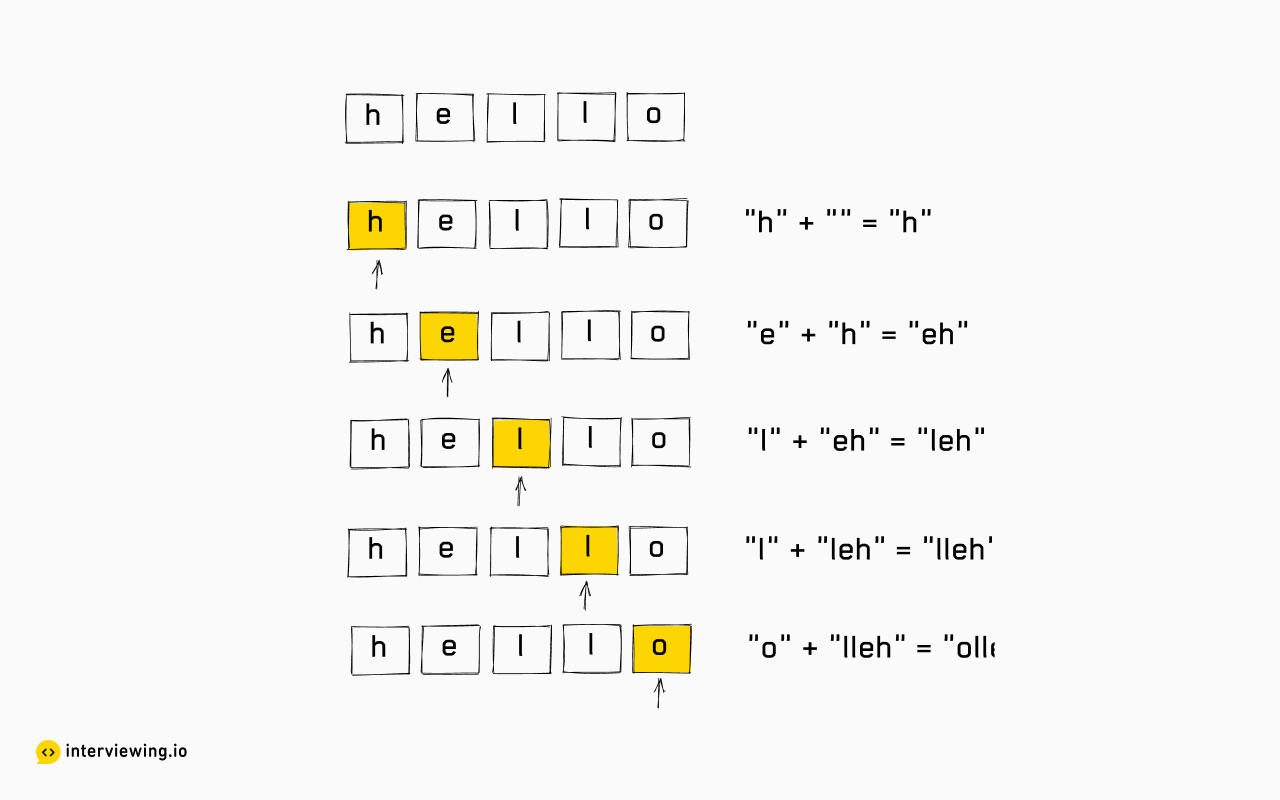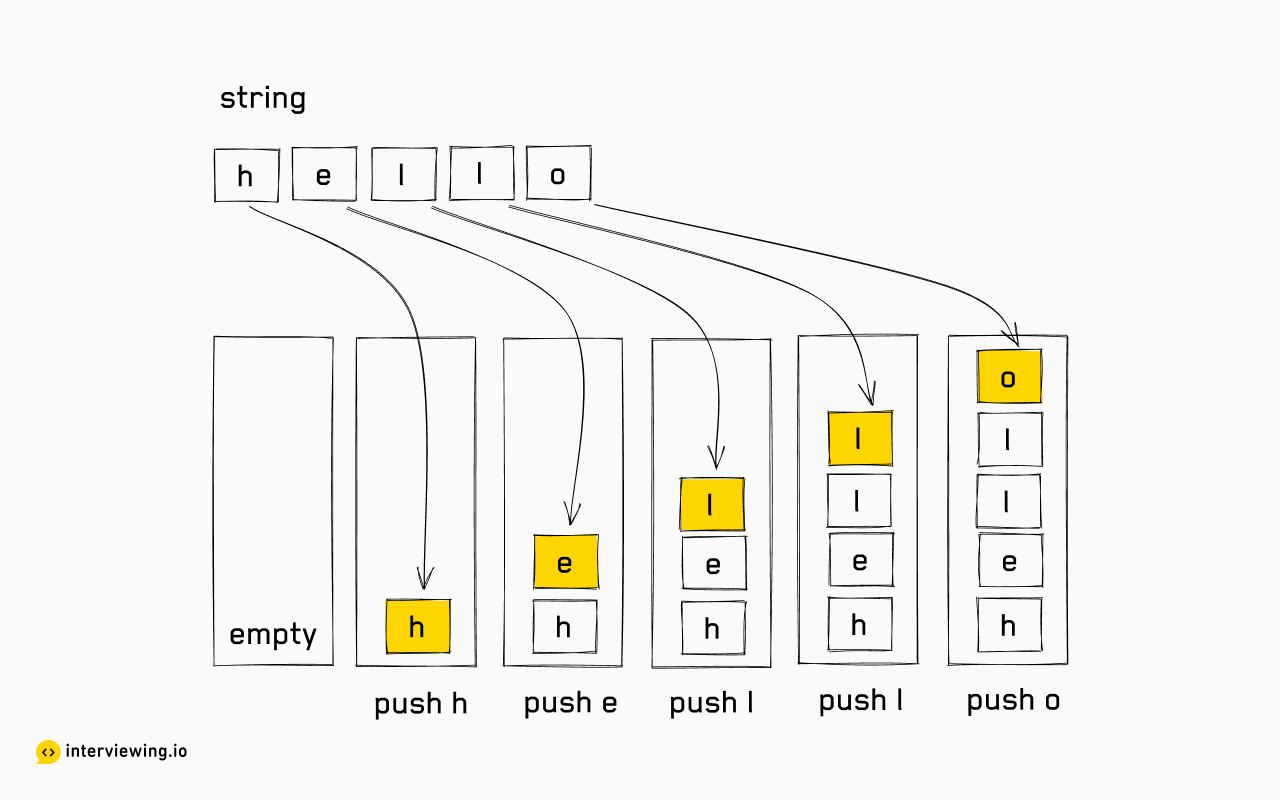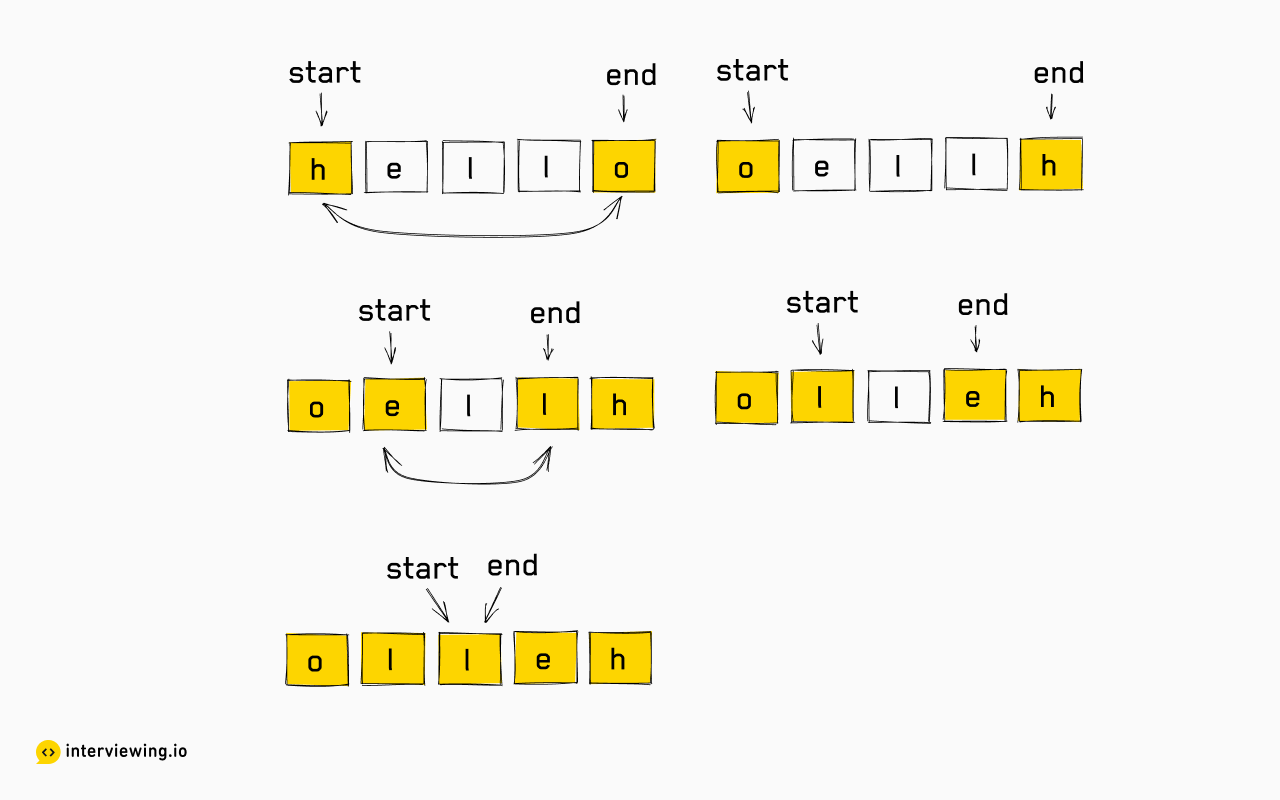EASY
DATA STRUCTURES AND ALGORITHMS

# How to Reverse a String (With Solutions in Python, Java & JavaScript)

Written ByTom WagnerJai Pandya

## How to Reverse a String: Problem Overview

The Reverse String problem involves taking a given string of characters and reversing the order of the characters. This problem, despite its simplicity, invites many advanced approaches, such iteration, recursion, or multiple pointers, each presenting a unique time and space complexity tradeoff.

## An Example of the Reverse String Problem

Write a program to reverse the given string. The program's output would be a string with all characters in reverse order.Input: "hello world" Output: "dlrow olleh"

Input: "aba" Output: "aba"

Input: "ab" Output: "ba"

Input: "" Output: ""

Constraints

The number of characters in the string would be in the range [0, 100000].

## How to Reverse a String : 4 Approaches in Python, Java & JavaScript

The most straightforward answer is to use an inbuilt library-provided function that is usually available in most languages. But the interviewer is probably not interested in our knowledge of library functions. This means the ideal solution would be similar to how a library would implement a `reverse` function (i.e., Python, JavaScript, Ruby). There are several approaches, and we'll discuss some of them here.

### Approach 1: Build String Iteratively (Brute Force)

The original string's first character turns into the reverse string's last character. The second character of the original string becomes the second last character of the reversed string. And so on.

We can loop through each character of the original string and build the reversed string iteratively. We start with an empty string and append the characters to it as we loop across the original string. Please note that we are appending the characters to the beginning of the string. By doing so, we ensure that the characters appearing later in the original string appear earlier in the reversed string.#### Algorithm

1. Initialize an empty string `reversed_string`.
2. Loop through each character of the original string.
3. Append the character at the beginning of `reversed_string`.
4. Return the `reversed_string`.

#### Reverse String JavaScript, Python and Java Solutions - Brute Force

``````function reverseString(string) {
let reversedString = "";
for (char of string) {
reversedString = char + reversedString;
}
return reversedString;
}``````

#### Time/Space Complexity

Let's assume that there are `n` characters in the given string.

• Time Complexity: `O(n²)`. A common mistake is to think of the time complexity of this approach as `O(n)`, but this is not the case. The time complexity is `O(n²)` because each time we append a character to the end of the string, we end up creating a new string. This new string then gets assigned to the variable `reversed_string`. This is an `O(n)` operation, and we are doing this for each character in the string. So the total time complexity is `O(n * n) = O(n²)`.

If we are coding in a language that support string mutability, then appending a character would be a constant time operation. So the time complexity would be `O(n)`.

• Space Complexity: `O(n)`. We are creating a new string of length `n`, which is the only memory space we use.

### Approach 2: Build String Iteratively (Linear Time)

In the previous approach, we noted that it was not the optimal solution because we were creating a new string each time we added a character to the end of the string, which is an `O(n)` operation. Can we find a way to bring this operation down to `O(1)`?

Instead of creating a new string every time we need to find a data structure that we can append individual characters to in constant time, and to this we can use a stack. A stack is a data structure that follows the LIFO principle. In this approach, we push all the characters of the original string onto the stack and then pop them one by one in order to build the reversed string.

Additionally, while building the reversed string, we use a dynamic array to store the characters. By using a dynamic array, we avoid creating a new string every time we append a character to the end of the string, making the operation `O(1)` instead of `O(n)`.#### Algorithm

1. Initialize an empty stack `stack`.
2. Loop through each character of the original string.
3. Push the character to the stack.
4. Initialize an empty dynamic array `reversed_string`.
5. Loop until the stack is empty.
6. Pop the top character from the stack. Append it to the end of `reversed_string`.
7. Create a string from the dynamic array `reversed_string` and return it.

#### Reverse String Javascript, Python and Java Solutions - Using a Stack

``````function reverseString(string) {
let stack = [];
for (char of string) {
stack.push(char);
}
let reversedString = [];
while (stack.length > 0) {
reversedString.push(stack.pop());
}
return reversedString.join("");
}``````

#### Time/Space Complexity

Let's assume that there are `n` characters in the given string.

• Time Complexity: `O(n)`. We are looping through the original string once and pushing the characters to the stack. Then we loop across the string again and pop all the characters from the stack. Finally, we join all the characters of reversed_string, so the total time complexity is `O(n + n + n) = O(3n) = O(n)` Please note that an algorithm that takes `3n` time is classified with `O(n)` time complexity. Big-O notation is a measure of how an algorithm scales as the size of the input grows. As the size of `n` increases, the constant factor `3` becomes insignificant.

• Space Complexity: `O(n)`, as we use a stack that stores `n` characters.

### Approach 3: In Place Reversal (Two Pointers)

When a string is reversed, the last character becomes the first character, and the first character becomes the last character. Similarly, the second last character becomes the second character, and the second character becomes the second last character. And so on. In other words, characters at the same position relative to the start and the end of the string are swapped.

We iterated from one end of the string to the other in the previous approaches. It is also possible to iterate from both ends of the string simultaneously. Let's have two pointers, one pointing at the start of the string and the other at the end of the string. We can swap the characters at these two pointers. Then we can move the pointers toward the middle of the string. We can keep doing this until the two pointers meet each other.

In most languages, a string is immutable. This means that we cannot change the characters of the string. We can only create a new string. So we need to convert the string to a character array. Then we can swap the characters at the two pointers. After swapping all the characters, we can transform the character array back to a string.

If the language supports mutable strings (e.g., Ruby, PHP, Swift), we can directly swap the characters at the two pointers. Some languages don't support mutability directly, but might have a class or a standard library that provides a mutable string. For example, there is StringBuilder in Java.#### Algorithm

1. Convert the string to a character array `char_array`.
2. Initialize two pointers, `start` and `end`, to point at the start and the end of the string, respectively.
3. Loop until `start` is less than `end`.
4. Swap the characters at `start` and `end`.
5. Increment `start` and decrement `end`.
6. Convert the character array back to a string and return it.

#### Reverse String Javascript, Python and Java Solutions - Using In Place Reversal

``````function reverseString(string) {
let charArray = string.split("");
let start = 0;
let end = charArray.length - 1;
while (start < end) {
swap(charArray, start, end);
start += 1;
end -= 1;
}
return charArray.join("");
}

function swap(charArray, start, end) {
// using destructuring assignment to swap the characters
[charArray[start], charArray[end]] = [charArray[end], charArray[start]];
}``````

#### Time/Space Complexity

Let's assume that there are `n` characters in the given string.

• Time Complexity: `O(n)`. Both pointers traverse the string from opposite ends until they merge. So every character is processed once in this process. Swapping two characters takes constant amount of time. So the overall time complexity is `O(n)`.

• Space Complexity: `O(n)`. We are using a character array to store the characters of the string. So the space complexity is `O(n)`. If the input to the function is a character array itself or the language supports string mutability, then the space complexity would be `O(1)`, because the algorithm does an in-place reversal of the character array / string.

### Approach 4: In Place Reversal (Recursion)

Note: While recursion will work for this problem, it overcomplicates the problem without added benefit and as such many interviewers will prefer one of the non-recursive approaches above. We have included the recursive solution here for completeness.

In the previous approach, we iterated two pointers toward the middle of the string and used them to swap the characters at the index of each pointer. While this method works well, we want to present a similar approach that uses recursion.

We define a recursive function that receives the character array and pointers for start and end as input. The function swaps the characters at the start and end pointers. Then it calls itself with the start pointer incremented by 1 and the end pointer decremented by 1. This way, the function keeps swapping the characters at the start and end pointers until the start pointer is greater than the end pointer. The function returns when the start pointer is greater than the end pointer. This way, we can reverse the string in place.

In the end, we convert the character array to a string and return it.

#### Algorithm

1. Convert the string to a character array `char_array`.
2. Call the recursive function `reverseStringHelper` with `char_array`, `0` and `char_array.length - 1` as input.
3. Convert the character array back to a string and return it.

Recursive function `reverseStringHelper`:

1. Base case: If `start` is greater than `end`, return.
2. Swap the characters at `start` and `end`.
3. Call the recursive function `reverseStringHelper` with `char_array`, `start + 1` and `end - 1` as input.

#### Reverse String Javascript, Python and Java Solutions - Using In Place Reversal with Recursion

``````function reverseString(string) {
let charArray = string.split("");
reverseStringHelper(charArray, 0, charArray.length - 1);
return charArray.join("");
}

function reverseStringHelper(charArray, start, end) {
if (start > end) {
return;
}
swap(charArray, start, end);
reverseStringHelper(charArray, start + 1, end - 1);
}

function swap(charArray, start, end) {
// using destructuring assignment to swap the characters
[charArray[start], charArray[end]] = [charArray[end], charArray[start]];
}``````

#### Time/Space Complexity

Let's assume that there are `n` characters in the given string.

• Time Complexity: `O(n)`. Both pointers traverse the string from opposite ends until they merge. We swap two characters in the main body of recursion, which is a constant time operation. So the overall time complexity is `O(n)`.

• Space Complexity: `O(n)`. We are using a character array to store the characters of the string. On top of that, we are using the call stack to store the function calls. We make `n/2` function calls, which is `O(n)`. So, the space complexity is `O(n)`.

## Practice the Reverse String Problem With Our AI Interviewer

Start AI Interview

## Reverse String Frequently Asked Questions (FAQ)

### Why can’t you just use reverse() when reversing a string?

In real life, you probably would. However, in an interview, you’ll want to demonstrate to your interviewer that you understand what programming languages do under the hood when they call a function like reverse(). That’s why it’s important to be able to implement it from scratch. It’s also an opportunity to demonstrate to your interviewer that you know if strings are mutable or immutable in your language of choice.

### How do you reverse a string in place?

To reverse a string in place means to modify the original string directly without using any additional memory. There are two ways to do this: the first is iterative, and the second uses recursion. Note that the recursive approach isn’t as efficient and overcomplicates the problem needlessly.

With the iterative approach, you’d use two pointers to swap characters symmetrically from both ends of the string: the first with the last, the 2nd with the 2nd to last, and so on. We’d repeat this approach until the two pointers met each other. Depending on whether strings are mutable or not in your programming language of choice, you might have to convert the string to a character array before doing the swaps.

With the recursive approach, we convert our string to a character array, and then pass it into the recursive function, along with pointers for the start and end. The function swaps the characters at the start and end pointers. Then it calls itself with the start pointer incremented by 1 and the end pointer decremented by 1. The function returns when the start pointer is greater than the end pointer.

### What’s the difference between reversing a string and reversing an array of integers?

How different these are depends on whether strings are mutable or not in your language of choice.

When reversing a string, you are dealing with a sequence of characters. Strings are typically treated as immutable in many programming languages, including Python, which means you cannot modify them directly. To reverse a string in place, you need to convert it into a mutable data structure (like an array) first, perform the reversal, and then convert it back to a string.

On the other hand, when reversing an array of integers, you are working with a collection of numeric values. Arrays of integers are mutable data structures in most programming languages, allowing direct modification. You can reverse the order of elements in an array by swapping elements at symmetric positions, without needing to convert the array into a different data type.